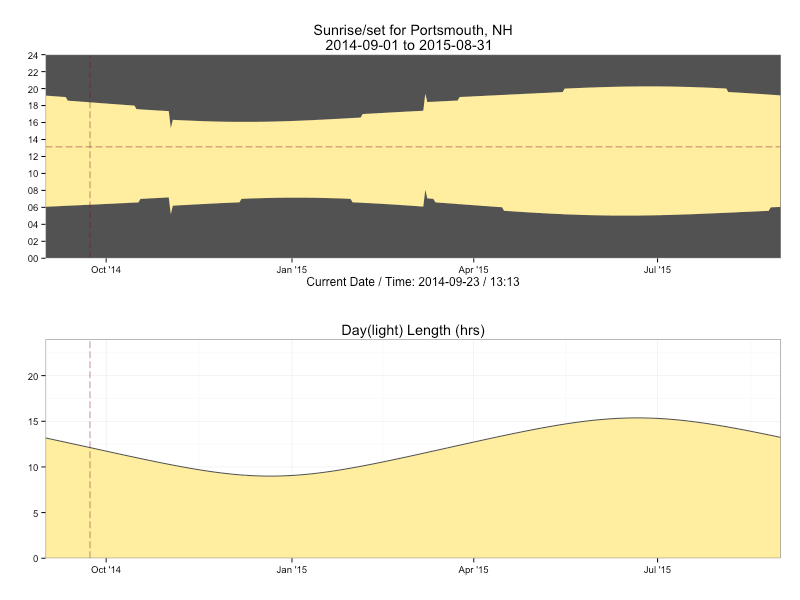Want to share your content on R-bloggers? click here if you have a blog, or here if you don't.

The arrival of the autumnal equinox foreshadows the reality of longer nights and shorter days here in the northeast US. We can both see that reality and distract ourselves from it at the same time by firing up RStudio (or your favorite editor) and taking a look at the sunrise & sunset times based on our map coordinates using some functions from the R maptools package.

The sunriset function takes in a lat/lon pair, a range of dates and whether we want sunrise or sunset calculated and returns when those ephemeral events occur. For example, we can see the sunrise time for Portsmouth, NH on Christmas day this year (2014) via:

 library(maptools)   # these functions need the lat/lon in an unusual format portsmouth <- matrix(c(-70.762553, 43.071755), nrow=1) for_date <- as.POSIXct("2014-12-25", tz="America/New_York") sunriset(portsmouth, for_date, direction="sunrise", POSIXct.out=TRUE)   ## day_frac time ## newlon 0.3007444 2014-12-25 07:13:04 

We can pass in a vector of dates, to this function, and that means we’ll have data points we can work with to visualize this change. Let’s wrap the sequence generation into a function of our own and extract:

• sunrise
• sunset
• solar noon
• # hours of daylight

for every day in the sequence, returning the result as a data frame.

 # adapted from http://r.789695.n4.nabble.com/maptools-sunrise-sunset-function-td874148.html ephemeris <- function(lat, lon, date, span=1, tz="UTC") {   # convert to the format we need lon.lat <- matrix(c(lon, lat), nrow=1)   # make our sequence - using noon gets us around daylight saving time issues day <- as.POSIXct(date, tz=tz) sequence <- seq(from=day, length.out=span , by="days")   # get our data sunrise <- sunriset(lon.lat, sequence, direction="sunrise", POSIXct.out=TRUE) sunset <- sunriset(lon.lat, sequence, direction="sunset", POSIXct.out=TRUE) solar_noon <- solarnoon(lon.lat, sequence, POSIXct.out=TRUE)   # build a data frame from the vectors data.frame(date=as.Date(sunrise$time), sunrise=as.numeric(format(sunrise$time, "%H%M")), solarnoon=as.numeric(format(solar_noon$time, "%H%M")), sunset=as.numeric(format(sunset$time, "%H%M")), day_length=as.numeric(sunset$time-sunrise$time))   } 

Now we can take a look at these values over 10 days near All Hallows Eve:

 ephemeris(43.071755, -70.762553, "2014-10-31", 10, tz="America/New_York")   ## date sunrise solarnoon sunset day_length ## 1 2014-10-31 716 1226 1736 10.332477 ## 2 2014-11-01 717 1226 1734 10.289145 ## 3 2014-11-02 518 1026 1533 10.246169 ## 4 2014-11-03 620 1126 1632 10.203563 ## 5 2014-11-04 621 1126 1631 10.161346 ## 6 2014-11-05 622 1126 1629 10.119535 ## 7 2014-11-06 624 1126 1628 10.078148 ## 8 2014-11-07 625 1126 1627 10.037204 ## 9 2014-11-08 626 1126 1626 9.996721 ## 10 2014-11-09 627 1126 1625 9.956719 

We now have everything we need to visualize the seasonal daylight changes. We’ll use ggplot (with some help from the grid package) and build a two panel graph, one that gives us a “ribbon” view of what hours of the day are in daylight and the other just showing the changes in the total number of hours of daylight available during the day. We’ll build the function so that it will:

• optionally show the current date/time (TRUE by default)
• optionally show when solar noon is (FALSE by default)
• optionally plot the graphs (TRUE by default)
• return an arrangeGrob of the charts in the event we want to use them in other charts
 library(ggplot2) library(scales) library(gridExtra)   # create two formatter functions for the x-axis display   # for graph #1 y-axis time_format <- function(hrmn) substr(sprintf("%04d", hrmn),1,2)   # for graph #2 y-axis pad5 <- function(num) sprintf("%2d", num)   daylight <- function(lat, lon, place, start_date, span=2, tz="UTC", show_solar_noon=FALSE, show_now=TRUE, plot=TRUE) {   stopifnot(span>=2) # really doesn't make much sense to plot 1 value   srss <- ephemeris(lat, lon, start_date, span, tz)   x_label = ""   gg <- ggplot(srss, aes(x=date)) gg <- gg + geom_ribbon(aes(ymin=sunrise, ymax=sunset), fill="#ffeda0")   if (show_solar_noon) gg <- gg + geom_line(aes(y=solarnoon), color="#fd8d3c")   if (show_now) { gg <- gg + geom_vline(xintercept=as.numeric(as.Date(Sys.time())), color="#800026", linetype="longdash", size=0.25) gg <- gg + geom_hline(yintercept=as.numeric(format(Sys.time(), "%H%M")), color="#800026", linetype="longdash", size=0.25) x_label = sprintf("Current Date / Time: %s", format(Sys.time(), "%Y-%m-%d / %H:%M")) }   gg <- gg + scale_x_date(expand=c(0,0), labels=date_format("%b '%y")) gg <- gg + scale_y_continuous(labels=time_format, limits=c(0,2400), breaks=seq(0, 2400, 200), expand=c(0,0)) gg <- gg + labs(x=x_label, y="", title=sprintf("Sunrise/set for %sn%s ", place, paste0(range(srss\$date), sep=" ", collapse="to "))) gg <- gg + theme_bw() gg <- gg + theme(panel.background=element_rect(fill="#525252")) gg <- gg + theme(panel.grid=element_blank())   gg1 <- ggplot(srss, aes(x=date, y=day_length)) gg1 <- gg1 + geom_area(fill="#ffeda0") gg1 <- gg1 + geom_line(color="#525252")   if (show_now) gg1 <- gg1 + geom_vline(xintercept=as.numeric(as.Date(Sys.time())), color="#800026", linetype="longdash", size=0.25)   gg1 <- gg1 + scale_x_date(expand=c(0,0), labels=date_format("%b '%y")) gg1 <- gg1 + scale_y_continuous(labels=pad5, limits=c(0,24), expand=c(0,0)) gg1 <- gg1 + labs(x="", y="", title="Day(light) Length (hrs)") gg1 <- gg1 + theme_bw()   if (plot) grid.arrange(gg, gg1, nrow=2)   arrangeGrob(gg, gg1, nrow=2)   } 

We can test our our new function using the same location and graph the sunlight data for a year starting September 1, 2014 (select graph for full-size version):

 daylight(43.071755, -70.762553, "Portsmouth, NH", "2014-09-01", 365, tz="America/New_York")With the longer nights approaching we can further enhance the plotting function to add markers for solstices and perhaps even make a new version that compares sunlight across different geographical locations.

Complete code example is in this gist.